# GED Math Practice Test 2

Continue your math review with our second free 2023 GED Math practice test. This math quiz is designed to help you prepare for the latest version of the GED Mathematical Reasoning test. Remember that you are allowed to use a calculator for these questions.

Directions: Solve each problem and select the best answer. You may use your calculator. You may also refer to the GED Formula Sheet as needed.

Congratulations - you have completed . You scored %%SCORE%% out of %%TOTAL%%. Your performance has been rated as %%RATING%%
 Question 1

### In Kingsport School, 46% of students are female. If the total number of students is 1250, then how many male students are attending this high school?

 A 698 B 692 C 687 D 675
Question 1 Explanation:
If 46% of the total number of students is female, then 100% − 46% = 54% of the students are male. Calculate 54% of the total to find the number of male students.

Recall that 54% is equivalent to 54 ÷ 100, or 0.54:
0.54 * 1250 = 675.
 Question 2

### Erin’s incomes and expenditures for the first five months of the year 2012 are given in the table below: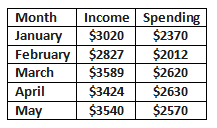### In which two months were her savings the highest?

 A January and February B February and March C March and May D January and March
Question 2 Explanation:
Erin’s monthly savings are found by calculating the difference between her income and her spending. Subtract each expenditure in the spending column from its corresponding income and find the two months that have the largest difference. Calculating the differences:

January: \$3020 − \$2370 = \$650
February: \$2827 − \$2012 = \$815
March: \$3589 − \$2620 = \$969
April: \$3424 − \$2630 = \$794
May: \$3540 − \$2570 = \$970

Erin saves the most during the months of March and May.
 Question 3

### Adding which number to the set will increase its mean?

 A 140 B 148 C 149 D 150
Question 3 Explanation:
The mean, or average of a data set, is found by adding the data points and dividing the sum by the number of data points. In order to increase the mean of a data set, a value larger than the mean must be added to the data set. Calculate the mean, and then find the answer choice that is larger than the mean:

Mean = (122 + 130 + 145 + 154 + 165 + 178) ÷ 6
= 894 ÷ 6
= 149

Of the choices available, only 150 will increase the mean.
 Question 4

### Each side of the figure below is 12 in. long. What is the area of this shape?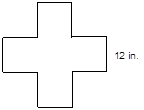A 5 ft2 B 6 ft2 C 12 ft2 D 36 ft2
Question 4 Explanation:
In cases like this, where there are multiple routes to the solution, it is best to first assess all of the information provided. You should notice that the shape’s side length is given in inches, but the answer choices are given in feet squared. As 12 inches are equivalent to 1 foot, and the shape is composed of 5 equivalent 1 foot by 1 foot squares, the answer must be 5 feet squared.
 Question 5

### Which of the following is equivalent to (7x + 3y)(8x + 5y)?

 A 7x² + 23xy + 5y² B 7x² + 24xy + 8y² C 56x² + 59xy + 15y² D 56x² + 35xy + 15y²
Question 5 Explanation:
Use the distributive property to multiply each term in the first parentheses through the second parentheses. When dealing with binomial expressions, this method is commonly referred to as the “FOIL method:” multiply the First two terms, then the Outer two terms, then the Inner two terms, and then the Last two terms, then combine like terms to arrive at the answer:

(7x + 3y)(8x + 5y)
56x² + 35xy + 24xy + 15y²
56x² + 59xy + 15y²
 Question 6

### A toy manufacturer makes 15,000 toys a year. The company randomly selects 300 of the toys to sample for inspection. The company discovers that there are 5 faulty toys in the sample. Based on the sample, how many of the 15,000 total toys are likely to be faulty?

 A 25 B 250 C 300 D 600
Question 6 Explanation:
The sample indicates that 5 out of every 300 randomly selected toys will be faulty. Consequently, a proportion can be set up that relates the unknown number of faulty toys in the total number of toys to the ratio of faulty toys to the sample. Stated otherwise:T is the unknown number of faulty toys in the total. Multiply both sides by 15,000 and then divide the left side by 300 to solve for T: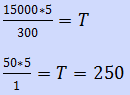Question 7

### Lisa earns \$8.10 an hour and worked 40 hours. Jamie earns \$10.80 an hour. How many hours would Jamie need to work to equal Lisa’s earnings over 40 hours?

 A 15 B 25 C 27 D 30
Question 7 Explanation:
Begin by calculating Lisa’s total earnings after 40 hours:
40 hours × \$8.10 an hour = \$324

Next, divide this total by Jamie’s hourly rate to find the number of hours Jamie would need to work:
\$324 ÷ \$10.80 = 30 hours
 Question 8

### If the sides of this cube are 4 inches long, what is the cube's volume?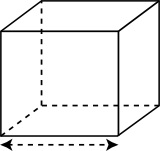A 64 in3 B 48 in3 C 32 in3 D 24 in3
Question 8 Explanation:
Use the formula for the volume of a cube and the given side length to calculate the cube’s volume.

V = s3 where s is side length
V = (4 in)3 = 64 in3
 Question 9

### Jenny is taking a vacation to Florida. She travels 70 kilometers per hour for 2 hours, and 63 kilometers per hour for 5 hours. Over the 7 hour time period what was Jenny’s average speed?

 A 64 km/h B 65 km/h C 66 km/h D 67 km/h
Question 9 Explanation:
In order to calculate the average speed over the entire trip, it is first necessary to determine the total distance traveled. Dividing the total distance traveled by the total time traveled then yields the average speed over the total trip. First calculate the distance she travels over the first 2 hours, recalling that distance is the product of rate and time: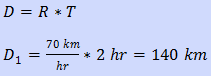Notice that dimensional analysis yields the appropriate units for distance (km). Next find the distance traveled over the last 5 hours of the trip:Her total distance is the sum of these distances:
D = D1 + D2 = 140 km + 315 km = 455 km.

Her average speed is her total distance divided by the total time traveled: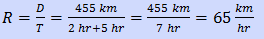Question 10

### Melody needs to calculate her monthly water bill. Her family used 18,900 gallons at a rate of \$1.12 per hundred gallons. There is also a monthly account fee of \$5.90 per household. What is her total bill?

 A \$205.90 B \$205.78 C \$211.68 D \$217.58
Question 10 Explanation:
Use dimensional analysis to combine the total number of gallons used with the cost per hundred gallons before adding this to the monthly account fee to find the total cost. Be careful with the conversion factor, as the cost is “per hundred gallons” and NOT “per gallon.” The setup is then: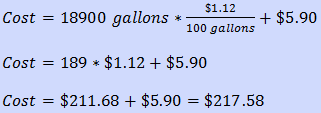Once you are finished, click the button below. Any items you have not completed will be marked incorrect.
There are 10 questions to complete.
 ← List →